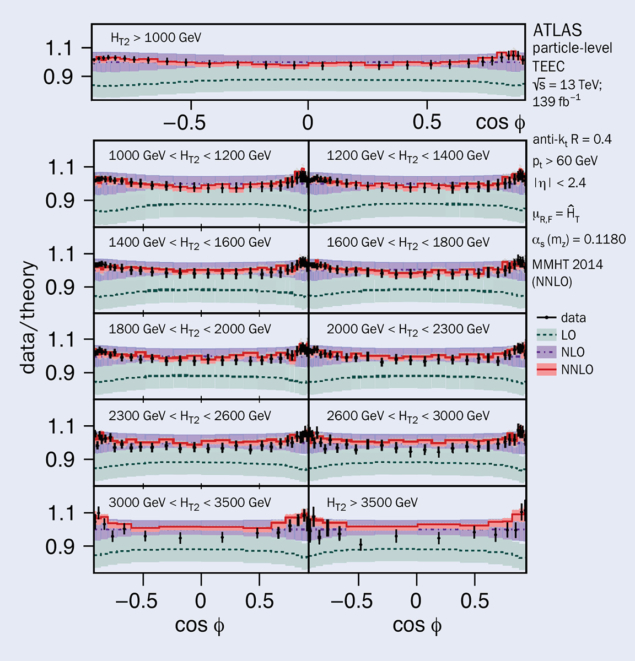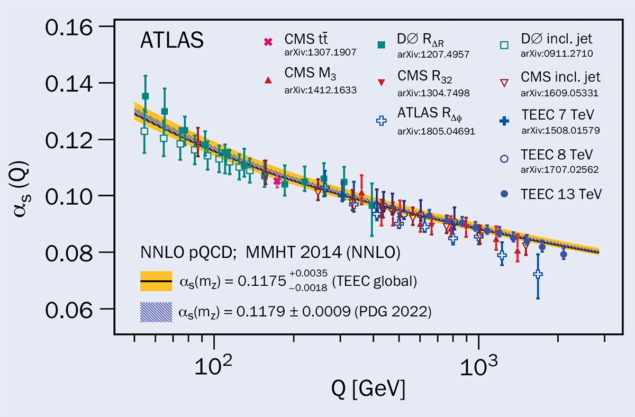# Strong coupling probed beyond the TeV scale

3 March 2023

A report from the ATLAS experiment.Fig. 1. The ratios of the theoretical predictions for the TEEC functions at leading order (green) and NNLO (red) in perturbative QCD to the NLO calculations (purple), together with the ratios of the data (black points) to NLO predictions. Source: arXiv:2301.09351

Due to asymptotic freedom, the theory of quantum chromodynamics (QCD), which describes the strong interaction acting on quarks and gluons, becomes weaker at short-distance (high-energy) scales. The value of the strong coupling constant αs at different scales can be determined using experimental observables that characterise the geometrical distribution of the outgoing hadrons from particle collisions. Among such observables are energy–energy correlations, which are obtained by computing the angular difference between all the possible pairs of final-state particles, weighted by the product of the normalised energies of the two particles involved.

### Precision tests

Energy–energy correlations had a significant impact on early precision tests of QCD, such as those performed at the PETRA e+e collider at DESY, where the gluon was discovered, and on the determination of αs. At hadron colliders such as the LHC, where the longitudinal momentum of the colliding partons is unknown, longitudinally invariant quantities are defined instead. The transverse energy–energy correlation (TEEC) function is defined as the transverse energy-weighted azimuthal-angular distribution of jet pairs, covering the full cos(φ) range, where φ is the azimuthal angular difference between the two jets. The TEEC distribution peaks at the edges of the cos(φ) range due to self-correlations, i.e. the angle between a jet and itself, and due to di-jet back-to-back configurations arising from momentum conservation between both jets in the transverse plane. Moreover, due to additional gluon radiation, a central plateau whose height is sensitive to the value of αs arises.

ATLAS has recently measured the TEEC distributions in multi-jet events and used them to determine αs. The analysis is performed using the full data sample (139 fb–1) of proton–proton collisions at a centre-of-mass energy of 13 TeV recorded during LHC Run 2. The measurements are presented in bins of the scalar sum of the transverse momenta of the two leading jets in the collision event (HT2), and are corrected for detector effects. By fitting theoretical calculations to the experimental data, the value of αs is determined in different kinematic regions, thereby testing the running of the strong coupling strength at high energy scales.

The results are 30% more precise than previous ATLAS measurements at 7 and 8 TeV, with a total systematic uncertainty of the order of 2.5% for the TEEC functions. The level of precision used for the theoretical predictions is equally important for the extraction of αs as that of the data. Finite predictions as a function of the value of αs(mZ), where mZ is the Z-boson mass, are calculated at next-to-next-to-leading order (NNLO) in perturbative QCD for three-jet configurations and corrected for non-perturbative effects, reducing the theoretical uncertainties in the central plateau from 6% at next-to-leading order (NLO) down to 2% for the NNLO predictions.Fig. 2. A comparison of the values of αs(Q), determined from fits to the TEEC functions with the QCD prediction using the world average (blue band) as input and the value obtained from the global fit (yellow band). The value of the momentum transfer Q is chosen as half of the average renormalisation scale, ĤT, in order to compare with results from previous measurements, where ĤT is the scalar sum of the transverse momenta of all final-state partons. Source: arXiv:2301.09351

The ratio of data to theoretical prediction for the TEEC functions in the various HT2 bins is shown in figure 1. The overall description of the shape at NNLO is found to be in agreement with the data, thus confirming our understanding of strong interactions over a large range of momentum transfers. Values of αs are determined from individual fits of the theoretical predictions to data in each HT2 bin. In addition, a global fit to the measured TEEC distributions results in αs(mZ) = 0.1175 ± 0.0001 (stat.) ± 0.0006 (syst.) +0.0034 –0.0017 (theo.), where the theoretical uncertainty is dominated by the scale uncertainties that estimate the contribution of higher-order corrections. All extracted values of αs agree with the recent world average.

This result represents the first αs determination with NNLO accuracy in three-jet production. Figure 2 compares the fitted values of αs with previous determinations from ATLAS and other experiments as a function of the momentum scale. The measurements clearly exhibit asymptotic freedom, and a significant improvement in precision compared to previous measurements.